## Extra Questions of Class 10 Science Chapter 2 Acids, Bases and Salts PDF Download

We have provided you with Extra and Important Questions from Class 10 Science Chapter 2 Acids, Bases, and Salts. This Extra and Important Questions will help you to score 100% in your Board Exams. These extra questions will be helpful to revise the important topics and concepts.

# Acids, Bases and Salts Class 10 Important Questions with Answers Science Chapter 2

## Extra Questions for Class 10 Science Chapter 2 Very Short Answer Type

Question: A metal carbonate X on reacting with an acid gives a gas which when passed through a solution Y gives the carbonate back. On the other hand, a gas G that is obtained at the anode during electrolysis of brine is passed on dry Y, it gives a compound Z, used for disinfecting drinking water. Identity X, Y, G and Z.   [NCERT Exemplar]
Calcium carbonate gives carbon dioxide gas when it reacts with hydrochloric acid.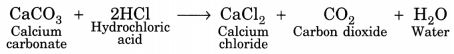Carbon dioxide turns lime water milky when passed through it because of formation of calcium carbonate. When carbon dioxide; so formed; is passed through lime water, it turns milky because of the formation of calcium carbonate.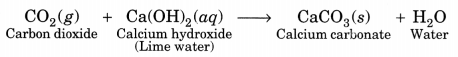On electrolysis of brine, chlorine gas is produced at the anode. Therefore G is Cl2.
Bleaching powder is used in disinfecting drinking water.
Therefore Z can be CaOCl2.
When chlorine is passed through dry calcium hydroxide [Ca(OH)2], bleaching powder CaOCl2 is formed.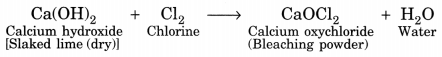Therefore, Y is calcium hydroxide, Ca(OH)2.
Since Y reacts with a gas to give a carbonate, the gas is CO2 and the carbonate is CaCO3.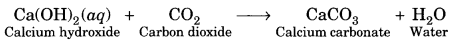Therefore, the metal carbonate X is calcium carbonate, CaCO3.
Therefore,

• Metal carbonate ‘X’ is calcium carbonate.
• Solution ‘Y is lime water (Calcium hydroxide).
• Gas ‘G’ is chlorine gas.
• Dry Y’ is dry calcium hydroxide (dry slaked lime).
• Compound ‘Z’ is bleaching powder (Calcium oxychloride).

Question: A substance X used as an antacid reacts with hydrochloric acid to produce a gas Y which is used in extinguishers.
(а) Name the substances X and Y.
(b) Write a balanced equation of the reaction between X and hydrochloric acid.   [CBSE 2011]
(a) Substance X is sodium hydrogencarbonate (X) and the gas evolved Y is carbon dioxide.
(b)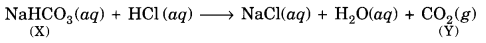Question: You are provided with the following materials in your laboratory:
Hydrochloric acid (HCl), sulphuric acid, (H2SO4), nitric acid (HNO3), acetic acid (CH3COOH), sodium hydroxide (NaOH), potassium hydroxide (KOH), calcium hydroxide [Ca(OH)2], magnesium hydroxide [Mg(OH)3] and ammonium hydroxide (NH4OH).
If we test each of the above solutions one by one with a drop of the following indicators, what colour change will you observe?
Red litmus, blue litmus, phenophthalein, methyl orange.
Acidic substances: HCl, H2SO4, HNO3, CH3COOH
They will turn blue litmus red and methyl orange red. There will be no effect on red litmus and phenolphthalein.

Basic substances: NaOH, KOH, Ca(OH)2, Mg(OH)2, NH4OH. They will turn red litmus blue and phenolphthalein pink. There will be no effect on blue litmus and methyl orange.

Question: The crystals of a compound A on keeping in air get converted into a white powder. Its solution in water gives blue colour with red litmus. It is used to remove permanent hardness from water.
(a) Identify the substance. Write chemical formula for its crystalline form.
(b) From the given information, identify the nature of the substance.
(c) Write two more uses of the substance.   [CBSE 2013]
(a) The substance is washing soda. Its chemical formula is Na2CO3.10H2O.
(b) Since the aqueous solution of the substance in water turns red litmus blue, it is of basic nature.
(c) (i) It is used in laundry for washing clothes.
(ii) It is used in the manufacture of glass, paper and chemicals like caustic soda (NaOH), and borax (Na2B4O7), etc.

Question: A substance X is used as a building material and is insoluble in water. When reacted with dilute HCl, it produces a gas which turns lime water milky. Predict the substance. Write the chemical equations evolved.
The substance is probably calcium carbonate (CaCO3), also called lime stone or marble. It is used as a building material. On reacting with dilute HCl, it evolves CO2 gas which turns lime water milky.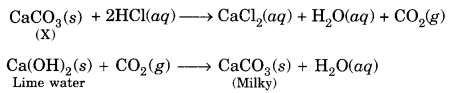Question: When electricity is passed through a common salt solution, sodium hydroxide is produced along with the liberation of two gases ‘X’ and T. The gas ‘X’ burns with a pop sound whereas T is used for disinfecting drinking water.  [CBSE 2011]
(i) Identify X and Y.
(ii) Give the chemical equation for the reaction stated above.
(iii) State the reaction of Y with dry slaked lime.
(i) The gas X’ is H2 and gas ‘Y’ is Cl2.
(ii) The chemical equation for the reaction is: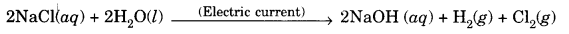(iii) Cl2 reacts with slaked lime to form bleaching powder.
Ca(OH)2 + Cl2 → CaOCl2 + H2O

Question: Which bases are called alkalies? Give an example of alkalies.

Answer: Soluble bases are called alkalies, e.g. sodium hydroxide (NaOH).

Question: Write a balanced chemical equation for the reaction between sodium carbonate and hydrochloric acid indicating the physical state of the reactants and the products.

Answer: Na2CO3(s) + 2HCl(aq) →  2NaCl(aq) + CO2(g) + H2O(l)

Question: Write a balanced chemical equation for a neutralisation reaction, mentioning the physical state of the reactants and the products.

Answer: NaOH(s) + 2HCl(aq) →  NaCl(aq) + H2O(l)

Question: What would be the colour of red litmus in a solution of sodium carbonate?

Answer: The red litmus will change to blue in sodium carbonate solution.

Question: What effect does an increase in concentration of H+(aq.) in a solution have on the pH of solution?

Answer: Higher the concentration, lower will be pH of the solution.

Question: Why does 1 M HC1 solution have a higher concentration of H+ ions than 1 M CH3COOH solution?

Answer: 1 M HCl has higher cone, of (H+) because it ionises completely in aqueous solution whereas CH3COOH does not as it is weak acid.

Question: Which gas is generally liberated when a dilute solution of hydrochloric acid reacts with an active metal?

Answer: Hydrogen gas is liberated when active metal reacts with dilute hydrochloric acid.

Zn(s) + 2HCl (dil.) → ZnCl2(aq) + H2(g)

Question: What is the colour of litmus in a solution of ammonium hydroxide?

Answer: Red litmus will turn blue in ammonium hydroxide.

Question: What happens when nitric acid is added to the egg shell?  [NCERT Exemplar]
Eggshell is made of calcium carbonate. When nitric acid is added to eggshell calcium nitrate, carbon dioxide and water are formed.
CaCO3 + 2HNO3 → Ca(NO3)2 + CO2 + H2O

Question: What is the concentration of H+ ion in pure water?
10-7

Question: Which one of these has a higher concentration of H+ ions? 1 M HCl or 1 M CH3COOH.   [CBSE 2009]
1 M HCl has higher concentration of H+ ions.

Question: Name an example of olfactory indicators.

Question: Name the chemical substance present in thick white and yellowish clouds present in the atmosphere of Venus.
Sulphuric acid.

Question:  A student detected the pH of four unknown solution A, B, C and D as follows 11, 5, 7 and 2. Predict the nature of the solution.

Answer: A is basic ‘B’ is acidic ‘C’ is natural and ‘D’ is strongly acidic.

Question:  State the chemical name of Plaster of Paris. Write a chemical equation to show the reaction between Plaster of Paris and water.

Question:  State in brief the preparation of washing soda from baking soda. Write balanced chemical equation of the reaction involved.

Answer: Sodium hydrogencarbonate (baking soda) on heating gives sodium carbonate which on recrystallisation gives washing soda.

Question: What is the colour of FeSO4.7H2O crystals? How does this colour change upon heating? Give balanced chemical equation for the changes.

Answer: Pale green is the colour of FeSO4.7H2O crystals. It becomes dirty white on heating.

Question:  Classify the following salts into acidic, basic and neutral: Potassium sulphate, ammonium chloride, sodium carbonate, sodium chloride.

Answer: Neutral: Potassium sulphate, Sodium chloride

Acidic: Ammonium chloride

Basic: Sodium carbonate

Question:  A student dropped few pieces of marble in dilute HC1 contained in a test tube. The evolved gas was passed through lime water.

(i) What change would be observed in lime water?

(ii) Write balanced chemical equation for the above change.

Answer: (i) Lime water will turn milky due to formation of calcium carbonate.

(ii) Ca(OH)2 (aq) + CO2 (g) → CaCO3 (s) + H2O(l)

Question:  What happens when chlorine is passed over slaked lime at 313K? Write chemical equation of the reaction involved and state two uses of the product obtained.

(i) It is used as bleaching agent in paper and textile industries.
(ii) It is used as disinfectant in purification of drinking water

## Extra Questions for Class 10 Science Chapter 2 Short Answer Type

Question How would you distinguish between baking powder and washing soda by heating?   [NCERT Exemplar]
Baking soda (NaHCO3) gives carbon dioxide and water vapour on heating at very low temperature. The gas so formed turns lime water milky, which confirms the presence of carbon dioxide gas.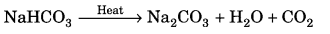When washing soda (Na2CO3) is heated it does not produce carbon dioxide even at high temperatures, but gives off its water of crystallisation to become anhydrous salt.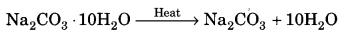Question A sulphate salt of Group 2 element of the Periodic Table is a white, soft substance, which can be moulded into different shapes by making its dough. When this compound is left in the open for some time, it becomes a solid mass and cannot be used for moulding purposes. Identify the sulphate salt and why does it show such a behaviour? Give the reaction involved.   [NCERT Exemplar]
Calcium belongs to group 2. Calcium sulphate is a white soft substance. It is known as Plaster of Paris, which can be moulded into different shapes by making its dough.

When Plaster of Paris is left for some time in the open, it turns into a solid mass because of reaction with moisture present in the atmosphere. The solid mass so formed is known as gypsum and cannot be further used for moulding.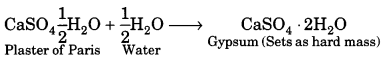The above said group 2 element is calcium sulpahte.

Question Name the acid present in ant sting and give its chemical formula. Also give the common method to get relief from the discomfort caused by the ant sting.   [NCERT Exemplar]
The acid present in ant sting: Methanoic acid
Chemical Formula of methanoic acid: HCOOH
Method to get relief from the discomfort caused by the ant sting: Rubbing baking soda over the area of ant sting.
Explanation: Rubbing baking soda (a base) over ant sting neutralises the methanoic acid present in the ant sting and gives relief from pain.

Question List two differences between acids and bases on the basis of chemical properties.
(i) Dilute acids like HCl and H2SO4 evolve H2 gas on reacting with metals like Zn, Mg and Ca, etc. and dilute bases do not evolve hydrogen gas.
(ii) Acids react with oxides of metals while bases react with oxides of non-metals.

Question List four main differences between acids and bases.

Question Mention the terms defined by the following sentences:
(a) A soluble base
(b) The insoluble solid formed when two solution are mixed together.
(a) Alkali
(b) Precipitate.

Question Name the product formed in each case when:
(а) hydrochloric acid reacts with caustic soda.
(b) granulated zinc reacts with caustic soda.
(a) The product formed is a mixture of sodium chloride and water.
NaOH (ag) + HCl (ag) → NaCl (ag) + H2O

(b) The product formed is a mixture of sodium zincate and hydrogen gas.
Zn (s) + 2NaOH (ag) → Na2ZnO2 (ag) + H2(g)

Question Explain why sodium hydroxide solution cannot be kept in aluminium containers? Write equation for the reaction that may take place for the same.
Sodium hydroxide solution reacts with aluminium to form sodium metaaluminate and hydrogen is evolved. Therefore, it cannot be kept in a container made of aluminium.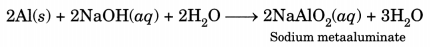Question How can you obtain the following gases by using dilute acid and one other substance?
(а) hydrogen
(b) carbon dioxide.
(a) Fe + H2SO4(dil.) → FeSO4 + H2(g)
Mg + 2HCl(dil.) → MgCl2 + H2(g)

(b) Na2CO3 + 2HCl(dil.) → 2NaCl + H2O + CO2(g)
NaHCO3 + HCl(dil.) → NaCl + H2O + CO2(g)

Question A solution of HCl is taken in a beaker and an electric circuit with a bulb is set up with the solution in series. What happens to the bulb and why?
The bulb will start glowing. Glowing of the bulb indicates that there is a flow of electric current through the solution. Electric current is carried through the solution by ions.

Since the cation present in acids is H+, this suggests that acids produce hydrogen ions, H+(ag), in solution, which are responsible for carrying current through the solution.

Question If 280 g of washing soda crystals are left in dry air for some time, a loss of weight of 162 g occurs. How can you account for this?
Washing soda (Na2CO3. 10H2O) is an efflorescent substance (if exposed to air, it loses most of its water of crystallisation). 280 g of washing soda lose 162 g of its water of crystallisation.

Question A sample of bleaching powder was kept in an air tight container. After a month, it lost some of its chlorine content. How will you account for it?
Bleaching powder if kept even in an air tight container, will slowly decompose on its own and form calcium chlorate and calcium chloride. The reaction is called auto oxidation. This will result in decrease in its chlorine contents.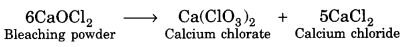Question A compound which is prepared from gypsum has the property of hardening when mixed with proper quantity of water. Identify the compound. Write chemical equation to prepare the compound. Mention one important use of the compound.
The compound is Plaster of Paris (CaSO4. $$\frac { 1 }{ 2 }$$ H2O). It is formed from gypsum (CaSO4 . $$\frac { 1 }{ 2 }$$ H2O) upon heating to a temperature of 373 K. It changes back to gypsum on adding water. Plaster of Paris is used for setting fractured bones.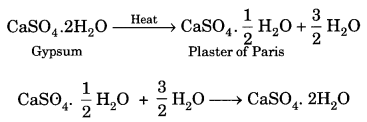Question A white powder is added while baking breads and cakes to make them soft and fluffy. Write the name of the powder. Name its main ingredients. Explain the function of each ingredient. Write the chemical reaction taking place when the powder is heated during baking.   [CBSE 2012, 2013]
The white powder is known as baking powder. The main ingredients are baking soda (NaHCO3) and tartaric acid (C4H6O6).

Question Explain giving reasons:
(i) Tartaric acid is a component of baking powder used in making cakes.
(ii) Gypsum (CaSO4. 2H2O) is used in the manufacture of cement.
(i) Role of tartaric acid in baking powder (mixture of tartaric acid and sodium hydrogencarbonate) is to neutralise sodium carbonate formed upon heating sodium hydrogencarbonate.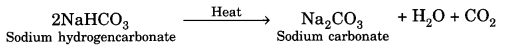In case it is not done, cake will be better and sodium carbonate will also have injurious side effects.

(ii) The role of gypsum (CaSO4.2H2O) in the manufacture of cement is to slow down the process of setting of cement.

## Extra Questions for Class 10 Science Chapter 2 Long Answer Type

Question What are strong and weak acids? In the following list of acids, separate strong acids from weak acids.  [NCERT Exemplar]
Hydrochloric acid, citric acid, acetic acid, nitric acid, formic acid, sulphuric acid.
Strong acid: Strong acids ionise completely in their aqueous solutions to produce a large number of hydrogen ions. Mineral acids are generally strong acids.

Weak acid: Weak acids do not ionise completely in their aqueous solution. Organic acids are generally weak acids.

Strong acid: Hydrochloric acid, nitric acid, sulphuric acid
Weak acid: Citric acid, acetic acid, formic acid

Question (i) Explain, why is hydrochloric acid a strong acid and acetic acid, a weak acid? How can it be verified?
(ii) Explain, why aqueous solution of an acid conducts electricity?
(iii) You have four solutions A, B, C and D. The pH of solution A is 6, B is 9, C is 12 and D is 7.
(a) Identify the most acidic and most basic solutions.
(b) Arrange the above four solutions in the increasing order of H+ ion concentration.
(c) State the change in colour of pH paper on dipping in solution C and D.   [CBSE 2012, 2013]
(i) Hydrochloric acid (HCl) is a stronger acid than acetic acid (CH3COOH) because it dissociates completely into H+ and Cl ions in aqueous solution. In order to verify this, add a few drops of universal indicator solution in the test tubes containing the acids. It acquires red colour in hydrochloric acid and yellow in acetic acid which confirms that hydrochloric acid is a stronger acid.

(ii) An aqueous solution of an acid releases ions in aqueous solutions. These ions conduct electricity.

(iii) (a) Most acidic is A (pH = 6) and most basic is C (pH = 12).
(b) The increasing order of H+ ion concentration is : C < B < D < A.
(c) The pH paper acquires dark purple colour in solution C and green in solution D.

Question Fill the missing data in the following table: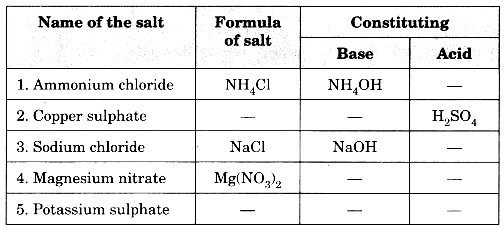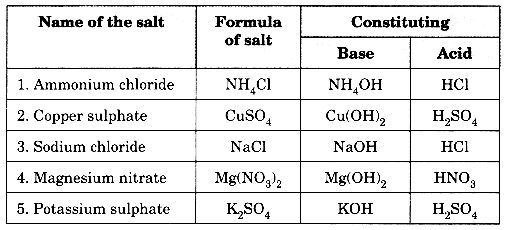Question (a) Explain how antacids give relief from acidity. Write the name of one such antacid.
(b) Fresh milk has a pH of 6. How does the pH change as it turns to curd? Explain your answer.
(c) A milkman adds a very small amount of baking soda to fresh milk. Why does this milk take a longer time to set as curd?
(d) Mention the nature of toothpastes. How do they prevent tooth decay?
(a) Our stomach produces hydrochloric acid that helps in digestion of food. During indigestion, our stomach produces excess acid. Antacids neutralise the excess of acid produced and gives relief from hyperacidity. Milk of magnesia (magnesium hydroxide) is one of such antacid.

(b) pH will decrease as it turns to curd because curd is acidic due to the presence of lactic acid.

(c) It takes longer time to set as curd as the presence of baking soda (sodium hydrogen carbonate) makes the milk basic and it does not allow it to become acidic easily.

(d) Toothpastes are basic in nature. They neutralise the acid formed in mouth which causes tooth decay.

Question (a) Explain the following chemical properties of acids with the help of balanced chemical equations only.
(i) when an acid reacts with a metal carbonate
(ii) when an acid reacts with a metal bicarbonate
(iii) when an acid reacts with a metal oxide.
(b) You are given three solutions A, B and C with pH values 2, 10 and 13 respectively. Write which solution has more hydrogen ion concentration among the three and state the nature ‘acidic or basic’ of each solution.